Introduction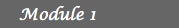1. The Binomial Model 2. Arbitrage opportunities 3. The First Fundamental  Theorem of Asset Pricing 4. Financial Derivatives 5. Hedging 6. Solutions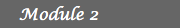7. Dividend payments 8. Families of risky assets 9. Time dependent interest  rates and rates of return 10. Short-selling prohibition 11. Multi-factor models 12. SolutionsThe First Fundamental Theorem of Asset Pricing

A first version of this theorem was proven by M. Harrison and D. Kreps in 1979. More general versions of the theorem were proven in 1981 by M. Harrison and S. Pliska and in 1994 by F. Delbaen and W. Schachermayer. For these extensions the condition of no arbitrage turns out to be too narrow and has to be replaced by a stronger assumption.
As we have seen in the previous lesson, proving that a market is arbitrage-free may be very tedious, even under very simple circumstances. In this lesson we will present the first fundamental theorem of asset pricing, a result that provides an alternative way to test the existence of arbitrage opportunities in a given market. When applied to binomial markets, this theorem gives a very precise condition that is extremely easy to verify (see Tangent).

Before stating the theorem it is important to introduce the concept of a Martingale. In simple words a martingale is a process that models a fair game. To make this statement precise we first review the concepts of conditional probability and conditional expectation.

## Note

We define in this section the concepts of conditional probability, conditional expectation and martingale for random quantities or processes that can only take a finite number of values. This turns out to be enough for our purposes because in our examples at any given time t we have only a finite number of possible prices for the risky asset (how many?). In more general circumstances the definition of these concepts would require some knowledge of measure-theoretic probability theory.
Conditional Probability
Given two events A and B such that P(A) (the probability of A) is not 0, the conditional probability of B given A is defined by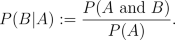This probability is interpreted as the probability of B given that A has occured. If the events A and B are independent we have that P(B|A)=P(B). For instance, in Example 3 of the previous lesson we have that the probability that the price of the stock goes up the second day given that it went down the first day is equal to P(price goes up the second day)=0.3.

Conditional Expectation
Once we have defined conditional probability the definition of conditional expectation comes naturally from the definition of expectation (see Probability review). Given a random variable or quantity X that can only assume the values x1, x2, ..., xn with possitive probability and an event A such that P(A) is not 0, the conditional expectation of X given A under the probability P is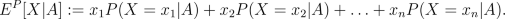For instance in Example 3 of the previous lesson we have that
EP (S2|S1 = 10*0.97)
= 10*0.97*1.01P(S2 = 10*0.97*1.01|S1 = 10*0.97) + 10*(0.97)2P(S2 = 10*(0.97)2|S1 = 10*0.97)
= 10*0.97*1.01*0.3 + 10*(0.97)2*0.7
= 9.5254.

Martingale
A random process X0, X1, ..., XT, such that for every t between 0 and T, Xt can only assume finitely many values xt1, ..., xtnt, is said to be a Martingale with respect to the probability measure P if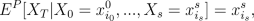for all s and i0, ..., is. In other words the expectation under P of the final outcome XT given the outcomes up to time s is exactly the value at time s. This happens if and only if for any t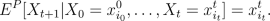Activity 1:
1. Suppose Xt is a gambler's fortune after t tosses of a "fair" coin (i.e P(heads)=P(tails)=0.5), where the gambler wins \$1 if the coin comes up heads and loses \$1 if the coin comes up tails. By using the definitions above prove that X is a martingale. This is also known as D'Alembert system and it is the simplest example of a martingale. It justifies the assertion made in the beginning of the section where we claimed that a martingale models a fair game.
2. Consider the market described in Example 3 of the previous lesson. Is the price process of the stock a martingale under the given probability?

## The Fundamental Theorem

A financial market with time horizon T and price processes of the risky asset and riskless bond given by S1, ..., ST and S10, ..., ST0, respectively, is arbitrage-free under the probability P if and only if there exists another probability measure Q such that
1. For any event A, P(A)=0 if and only if Q(A)=0. We say in this case that P and Q are equivalent probability measures.
2. The discounted price process, X0:=S0/S00, ..., XT:=ST/S0T is a martingale under Q.
A measure Q that satisifies (i) and (ii) is known as a risk neutral measure. After stating the theorem there are a few remarks that should be made in order to clarify its content. The first of the conditions, namely that the two probability measures have to be equivalent, is explained by the fact that the concept of arbitrage as defined in the previous lesson depends only on events that have or do not have measure 0. More specifically, an arbitrage opportunity is a self-finacing trading strategy such that the probability that the value of the final portfolio is negative is zero and the probability that it is positive is not 0, and we are not really concerned about the exact probability of this last event. Also notice that in the second condition we are not requiring the price process of the risky asset to be a martingale (i.e a fair game) but the discounted price process. This can be explained by the following reasoning: suppose that the price of the risky asset is measured in dollars. If at time t the price of the risky asset is \$x, the fair price the seller should charge at time 0 for the asset should be \$x/(1+r)t, with r the interest rate. If he charges \$y<\$x/(1+r)t then the buyer could take advantage of the situation by borrowing \$y at time 0 to buy the asset and then selling at time t to repay his debt of \$y(1+r)t, obtaining a positive profit of \$(x-y(1+r)t). If he charges \$y>\$x/(1+r)t then he could take advange of the situation by selling the asset at time 0 and lending \$y dollars so that at time t he would receive \$y(1+r)t and after buying back the asset he would make a positive profit of \$(y(1+r)t-x). Basically when one considers the discounted price process every price is being measured in the unity of the riskless asset S0, which is also commonly known in the literature as the Numéraire.

Activity 2: Application of the FFTAP to the CRR Model
Consider a CRR Model with interest rate r. Assume that at any time t the return rate of the risky asset R satisifes P(Rt=b)=p and P(Rt=a)=1-p with p strictly greater than 0 and strictly less than 1. A binary tree structure of the price process of the risly asset is shown below.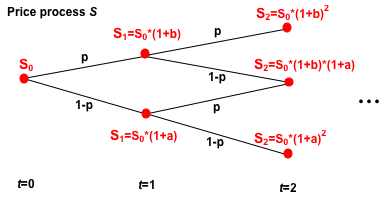1. Explain why a probability measure Q such that at any time t the return rate of the risky asset, R, satisifes Q(Rt=b)=q and Q(Rt=a)=1-q and Rt+1,Rt are independent, is equivalent to P if and only if q is strictly between 0 and 1.
2. Suppose that your time horizon is T=1 and that Q* is a measure. Prove that the discounted price process is a martingale under Q* if and only if at any time t the return rate of the risky asset R satisifes Q*(Rt=b)=(r-a)/(b-a) and Q*(Rt=a)=(b-r)/(b-a).
3. Extend the previous reasoning to an arbitrary time horizon T. Hint: The discounted price process X is a maritngale under Q* if and only if for any time t greater than 0, EQ*[Xt|Xt-1=x]=x.
4. Explain why this CRR model is arbitrage free if and only if a < r < b. In this case the only risk neutral measure is given by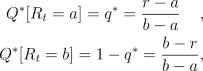for any time t. Hint: Recall that the probability of an event must be a number between 0 and 1.
5. What happens if p=0 or p=1?
6. Verify the result given in d) for the Examples given in the previous lessons.
Important note: The justification of each of the steps above does not have to be necessarily formal. A more formal justification would require some background in mathematical proofs and abstract concepts of probability which are out of the scope of these lessons. However, the statement and consequences of the First Fundamental Theorem of Asset Pricing should become clear after facing these problems.